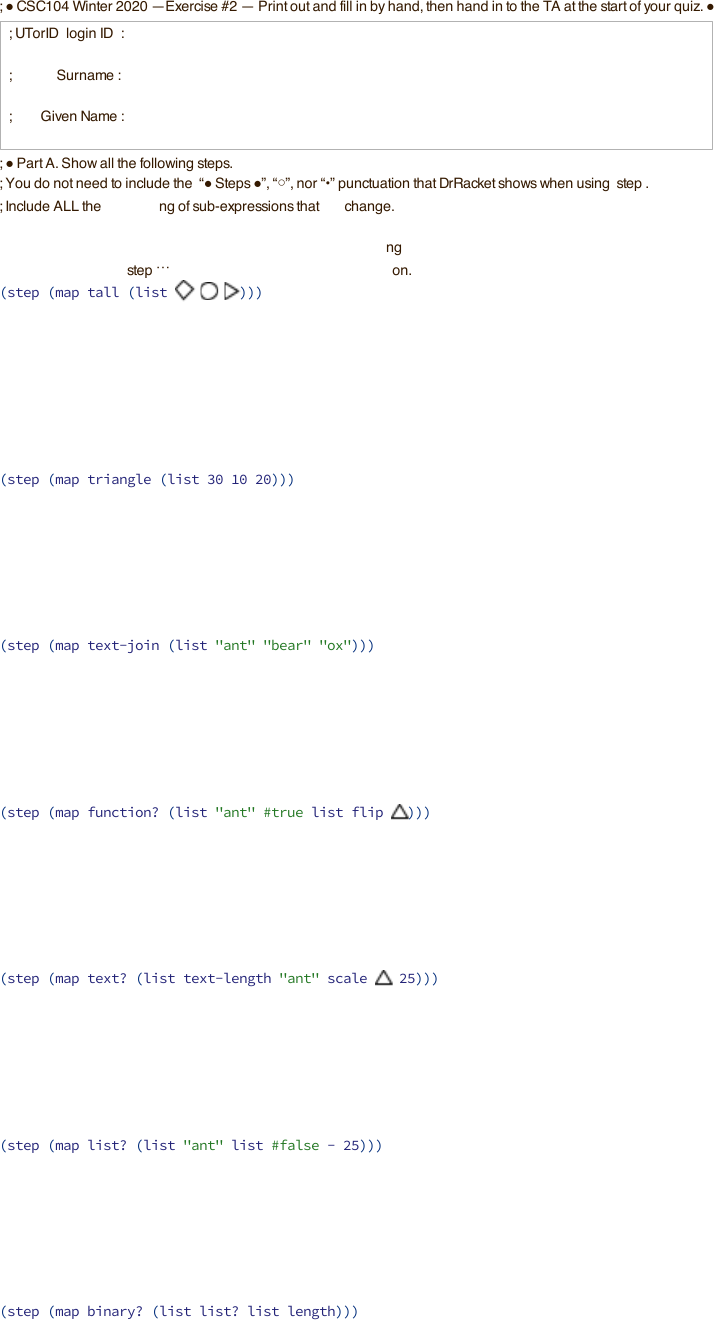Study Guides (400,000)
CA (150,000)
UTSG (10,000)
CSC (300)
Quiz

# CSC104H1 Quiz: exercise-2Exam

Department
Computer Science
Course Code
CSC104H1
Professor
Gary Baumgartner
Study Guide
Quiz

This preview shows page 1. to view the full 4 pages of the document.;
!
C
S
C
1
0
4
W
i
n
t
e
r
2
02
0
E
x
e
r
c
i
s
e
#
2
P
r
i
n
t
o
u
t
a
n
d
f
i
l
l
i
n
b
y
h
a
n
d
,
t
h
e
n
h
a
n
d
i
n
t
o
t
h
e
T
A
a
t
t
h
e
s
t
a
r
t
o
f
y
o
u
r
q
u
i
z
.
!
;
U
T
o
r
I
D
(
l
o
g
i
n
I
D
)
:
;
S
u
r
n
a
m
e
:
;
G
i
v
e
n
N
a
m
e
:
; !
r
t
A
.
S
h
o
w
a
l
l
t
h
e
f
o
l
l
o
w
i
n
g
s
t
e
p
s
.
;
Y
o
u
d
o
n
o
t
n
e
e
d
t
o
i
n
c
l
u
d
e
t
h
e
!
S
t
e
p
s
!
,
,
n
o
r
p
u
n
c
t
u
a
t
i
o
n
t
h
a
t
D
r
R
a
c
k
e
t
s
h
o
w
s
w
h
e
n
u
s
i
n
g
s
t
e
p
.
;
I
n
c
l
u
d
e
A
L
L
t
h
e
underlining
of
s
u
b
-
e
x
p
r
e
s
s
i
o
n
s
that
will change.
; In DrRacket, the step operation starts by copying the expression given to step so that it can add the underlining
; for
that initial expression, but you may save some effort
by
the initial underlining directly to the
original
; expression inside
(
step
) rather than recopying that expression.
(
s
t
e
p
(
m
a
p
t
a
l
l
(
l
i
s
t
)
)
)
(
step
(
map
t
r
i
a
n
g
l
e
(
list
3
0
1
0
2
0
)))
(
step
(
map
t
e
x
t
-
j
o
i
n
(
list
"
a
n
t
"
"
b
e
a
r
"
"ox"
)))
(
step
(
map
f
u
n
c
t
i
o
n
?
(
list
"ant"
#
t
r
u
e
list
flip
)))
(
step
(
map
text?
(
list
t
e
x
t
-
l
e
n
g
t
h
"ant"
scale
2
5
)))
(
step
(
map
list?
(
list
"ant"
list
#false
-
25
)))
(
step
(
map
b
i
n
a
r
y
?
(
list
list?
list
length
)))